# Pentagonal polytope

In geometry, a pentagonal polytope is a regular polytope in n dimensions constructed from the Hn Coxeter group. The family was named by H. S. M. Coxeter, because the two-dimensional pentagonal polytope is a pentagon. It can be named by its Schläfli symbol as {5, 3n 2} (dodecahedral) or {3n 2, 5} (icosahedral).

## Family members

The family starts as 1-polytopes and ends with n = 5 as infinite tessellations of 4-dimensional hyperbolic space.

There are two types of pentagonal polytopes; they may be termed the dodecahedral and icosahedral types, by their three-dimensional members. The two types are duals of each other.

### Dodecahedral

The complete family of dodecahedral pentagonal polytopes are:

1. Line segment, { }
2. Pentagon, {5}
3. Dodecahedron, {5, 3} (12 pentagonal faces)
4. 120-cell, {5, 3, 3} (120 dodecahedral cells)
5. Order-3 120-cell honeycomb, {5, 3, 3, 3} (tessellates hyperbolic 4-space (∞ 120-cell facets)

The facets of each dodecahedral pentagonal polytope are the dodecahedral pentagonal polytopes of one less dimension. Their vertex figures are the simplices of one less dimension.

Dodecahedral pentagonal polytopes
n Coxeter group Petrie polygon
projection
Name
Coxeter diagram
Schläfli symbol
Facets Elements
Vertices Edges Faces Cells 4-faces
1[ ]
(order 2)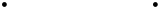Line segment{ }
2 vertices 2
2(order 10)Pentagon{5}
5 edges 5 5
3[5,3]
(order 120)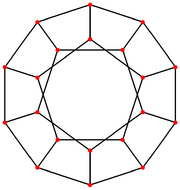Dodecahedron{5, 3}
12 pentagons20 30 12
4[5,3,3]
(order 14400)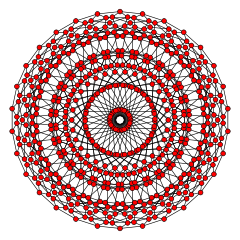120-cell{5, 3, 3}
120 dodecahedra600 1200 720 120
5[5,3,3,3]
(order ∞)
120-cell honeycomb{5, 3, 3, 3}
120-cells### Icosahedral

The complete family of icosahedral pentagonal polytopes are:

1. Line segment, { }
2. Pentagon, {5}
3. Icosahedron, {3, 5} (20 triangular faces)
4. 600-cell, {3, 3, 5} (120 tetrahedron cells)
5. Order-5 5-cell honeycomb, {3, 3, 3, 5} (tessellates hyperbolic 4-space (∞ 5-cell facets)

The facets of each icosahedral pentagonal polytope are the simplices of one less dimension. Their vertex figures are icosahedral pentagonal polytopes of one less dimension.

Icosahedral pentagonal polytopes
n Coxeter group Petrie polygon
projection
Name
Coxeter diagram
Schläfli symbol
Facets Elements
Vertices Edges Faces Cells 4-faces
1[ ]
(order 2)Line segment{ }
2 vertices 2
2(order 10)Pentagon{5}
5 Edges 5 5
3[5,3]
(order 120)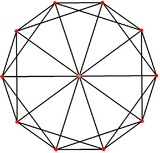Icosahedron{3, 5}
20 equilateral triangles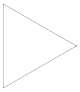12 30 20
4[5,3,3]
(order 14400)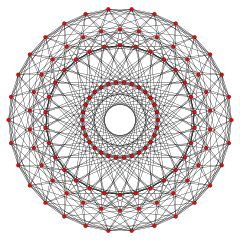600-cell{3, 3, 5}
600 tetrahedra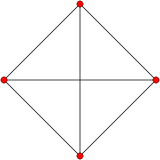120 720 1200 600
5[5,3,3,3]
(order ∞)
Order-5 5-cell honeycomb{3, 3, 3, 5}
5-cells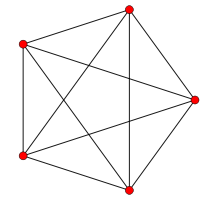## Related star polytopes and honeycombs

The pentagonal polytopes can be stellated to form new star regular polytopes:

## References

• Kaleidoscopes: Selected Writings of H.S.M. Coxeter, edited by F. Arthur Sherk, Peter McMullen, Anthony C. Thompson, Asia Ivic Weiss, Wiley-Interscience Publication, 1995, ISBN 978-0-471-01003-6
• (Paper 10) H.S.M. Coxeter, Star Polytopes and the Schlafli Function f(α,β,γ) [Elemente der Mathematik 44 (2) (1989) 2536]
• Coxeter, Regular Polytopes, 3rd. ed., Dover Publications, 1973. ISBN 0-486-61480-8. (Table I(ii): 16 regular polytopes {p, q,r} in four dimensions, pp. 292293)
Fundamental convex regular and uniform polytopes in dimensions 2–10
Family An Bn I2(p) / Dn E6 / E7 / E8 / E9 / E10 / F4 / G2 Hn
Regular polygon Triangle Square p-gon Hexagon Pentagon
Uniform polyhedron Tetrahedron OctahedronCube Demicube DodecahedronIcosahedron
Uniform 4-polytope 5-cell 16-cellTesseract Demitesseract 24-cell 120-cell600-cell
Uniform 5-polytope 5-simplex 5-orthoplex5-cube 5-demicube
Uniform 6-polytope 6-simplex 6-orthoplex6-cube 6-demicube 122221
Uniform 7-polytope 7-simplex 7-orthoplex7-cube 7-demicube 132231321
Uniform 8-polytope 8-simplex 8-orthoplex8-cube 8-demicube 142241421
Uniform 9-polytope 9-simplex 9-orthoplex9-cube 9-demicube
Uniform 10-polytope 10-simplex 10-orthoplex10-cube 10-demicube
Uniform n-polytope n-simplex n-orthoplexn-cube n-demicube 1k22k1k21 n-pentagonal polytope
Topics: Polytope familiesRegular polytopeList of regular polytopes and compounds